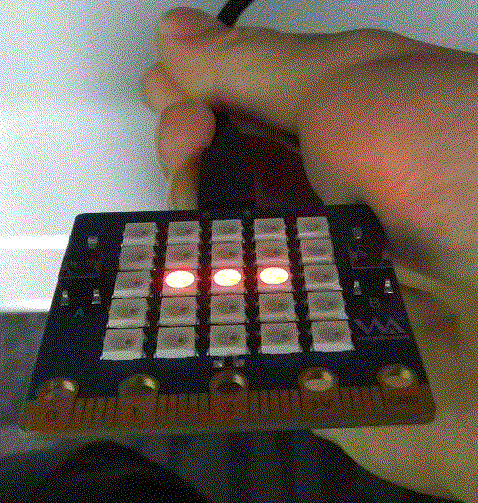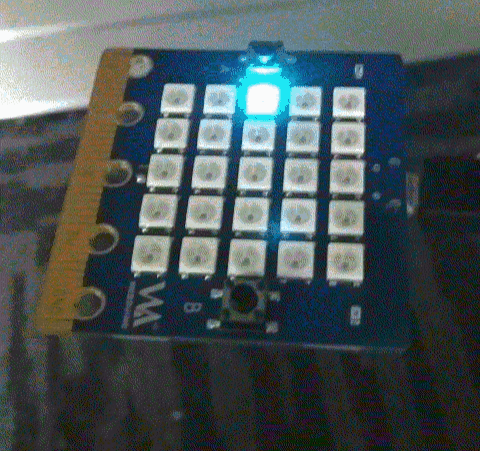# 10. 板子姿态检测¶

## 10.1. 先体验试一试¶

```from microbit import *

while True:
reading = accelerometer.get_x()
if reading > 20:
display.show("R")
elif reading < -20:
display.show("L")
else:
display.show("-")
```## 10.2. 反馈板子姿态¶

```from microbit import *

while True:
gesture = accelerometer.current_gesture()
if gesture == "face up":
display.show(Image.HAPPY)
else:
display.show(Image.ANGRY)
```

## 10.3. 体验平衡球游戏¶

```import utime
from random import randint
from machine import I2C, Pin
from mpu9250 import MPU9250

i2c = I2C(scl=Pin(22), sda=Pin(21), freq=200000)
sensor = MPU9250(i2c)
print("MPU9250 id: " + hex(sensor.whoami))
from display import Pixel, PixelPower

PixelPower(True)
View = Pixel()
X, Y, Color, Flag = 2, 2, 2, 0
while True:
# print('acceleration:', sensor.acceleration)
# print('gyro:', sensor.gyro)
# print('magnetic:', sensor.magnetic)
A = sensor.acceleration  # -1 and -2 Software correction
View.LoadXY(X, Y, (0, 0, 0), False)
if (A > -1 and A > X and X < View.Max - 1):
X = X + 1
elif (A < -1 and A < X and X > View.Min):
X = X - 1
if (A > -2 and A > Y and Y > View.Min):
Y = Y - 1
elif (A < -2 and A < Y and Y < View.Max - 1):
Y = Y + 1

Color = Color + Flag
if (Color == 10):
Flag = -2
elif (Color == 2):
Flag = +2

View.LoadXY(X, Y, (0, Color, Color), False)
View.Show()
utime.sleep_ms(100)
```